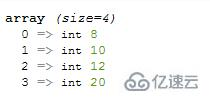# php如何给数组增加一个数组元素php给数组增加一个数组元素的方法

1、array_unshift()函数在数组中插入新元素

`array_unshift(\$array,\$value1,\$value2...)`函数可以在数组的开头插入一个或多个新元素（键值）。

```<?php
\$arr=array(10,12,20);
array_unshift(\$arr,8);
var_dump(\$arr);
?>```array_unshift()函数不会保持原有的数值索引关系，会删除所有的数值键名，并为其重新赋值，即从 0 开始重新计数；但所有的字符串键名保持不变。

```<?php
\$arr=array("a"=>"red","b"=>"green",3=>"pink");
echo "原来的数组：";
var_dump(\$arr);
array_unshift(\$arr,"blue");
echo "在开头插入一个新元素后：";
var_dump(\$arr);
?>```2、array_push()函数在数组中插入新元素

`array_push(\$array,\$value1,\$value2...)`函数可以在数组尾部插入一个或多个元素（键值）。

```<?php
\$arr=array(10,12,20);
array_push(\$arr,8);
var_dump(\$arr);
?>```array_push()函数和array_unshift()函数不一样，它不会重置数值键名，而是在原有数值键名的基础上计数。

```<?php
\$arr=array("a"=>"red","b"=>"green",3=>"pink");
array_push(\$arr,8,"9",3.14);
var_dump(\$arr);
?>````array_pad(\$array,\$size,\$value)`函数可以将某个键值`\$value`插入到数组`\$array`中，从而将数组填补到指定的长度`\$size`。（`\$size`参数可以理解为数组中元素的最终数目，即插入操作后的数组长度）。

```<?php
\$arr=array(10,12,20);
var_dump(\$result);
?>````\$size`参数有三种取值情况：

• 为正数，则在数组尾部插入元素；

• 为负数，则在数组的开头插入元素；

• 如果其绝对值小于等于 `\$array` 数组的长度，则不进行插入操作。

```<?php
\$arr=array(10,12,20);
var_dump(\$result);
var_dump(\$result);
var_dump(\$result);
?>``````<?php
\$arr=array(10,12,20);